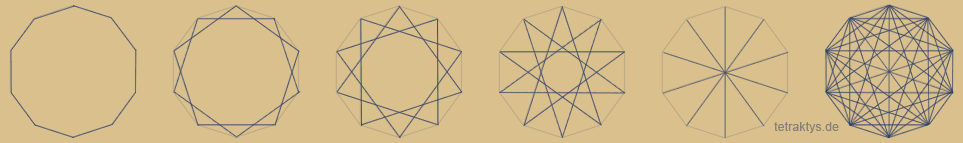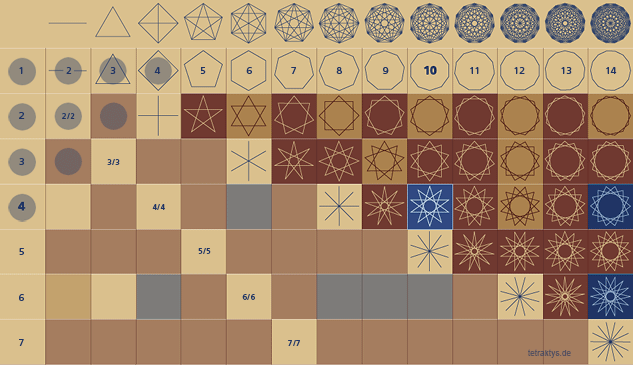# Pythagorean Tetraktys

In the Wikipedia entry for Tetraktys (as of July 2016), the sub-item „Ancient meaning“ is introduced with: „As Tetraktys the Pythagoreans called the set of numbers 1, 2, 3 and 4, the sum of which gives 10.“

Hans Kayser had a different opinion on this topic. In a correspondence to a friend he writes: „This quadrant is always denoted by 1 + 2 + 3 + 4 = 10, which was calculated only for the stupid ones.6, 8, 9, 12, however, combines the arithmetic, geometric and harmonic proportions in itself, that is the order structure of the world at that time „. (Quoted from the booklet „The Mystery of the Pythagorean Tetraktys“ by Theo Reiser from 1967.

Much has been asserted and speculated about concrete details of the so-called Tetraktys = Quaternary of the Pythagoreans. Both in the corresponding Wikipedia entry and elsewhere – including on the Website tetraktys.de – you will find the range of significant quaternary more or less completely listed. Of course, this raises the question of what exactly corresponds to the truth.

In the search for relevant answers, however, you cannot ignore exactly two ancient sources. These are, on the one hand, the fragments of the Pythagorean Philolaos. In it one finds among other things the following Statement:

„You have to know the achievements and the essence of the number
according to the force, which is in the ten-number.
Because great and perfect
and effecting everything
and divine and heavenly
as well as human life beginning
as well as participating leader is the force
of the number and the ten.
Because without them everything is unlimited
and indistinct and unclear „

Furthermore, the attentive reader will notice a text passage of an ancient book entitled „From the Pythagorean Numbers,“ which appears and is quoted in various fragmentary collections of the pre-Socratics. The content of this book is also according to matching sources, also from Philola itself. It was written by Speusippos, the son of Plato’s sister.

From this text it emerges what fascinated the Pythagoreans in the crowd 10 so that all possible divisor properties are included for the first time in the „ten number“. We are talking about the „first and unconnected“ and the „second and compound“. What is that supposed to mean? Not without the appropriate geometry. If you represent sets of numbers as polygons and star polygons, you can see that the 3 or 4 divisor properties of sets are also assigned to 4 types of polygons and star polygons, here directly on the example of the 10-corner:Furthermore, the even numbers are referred to as „the closing numbers“. What is that supposed to mean? Not without the appropriate geometry. Even-numbered polygons complete with the inside angle sum of circles, while odd numbers begin with a half-circle (new vertex = triangle of 180 degrees).

The extant text of this book „From the Pythagorean Numbers“ can be found  here
Similarly, the description of the geometric equivalent.

What makes this issue so harmonious in terms of harmonics?
Now:

All these divisional properties of sets, represented as star polygons, can be found in an interesting „rotation matrix“ in the most important diagram of the harmonics, the Lambdoma. The resulting pattern corresponds to the representation of physical waves. The equal-harmonics of Hans Kayser’s „harmonic tetractices“, ie 6, 8, 9, 12 and 1/2, 2/3, 3/4, correspond to the representation of point, line, triangle and square within this matrix. So 1 + 2 + 3 + 4. This closes the harmonic circle.

Another interesting aspect of this topic is the fact that many polygons and star polygons have assumed an important symbolic meaning from antiquity through the Renaissance to the present day. The embedding in a stringent and complex system such as the Lambdoma or the division table thus allows detailed statements about the respective symbol on a scientifically sound Basis.

Exemplary for this approach is the pentagram, as a distinctive mark of the Pythagoreans, which is to be found as a „double pentagram“ for the first time as the third and last divisor in the constellation 10/4, see the following figure:The double pentagram, ie the upright and the fallen pentagram symbolizes the human in matter, as well as the square with the cross and its center – that is 10 divided by 4.

More Details can be found on the websiteof Hoger Ullmann: tetraktys.de

An expanded essay on this subject (in German) can be found on this Website as pdf-date here.